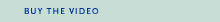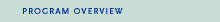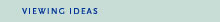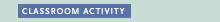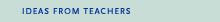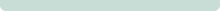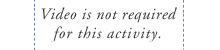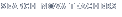Back to Teachers HomeSecrets of Lost Empires I—ObeliskClassroom ActivityObjective
To investigate how levers can be used to lift objects.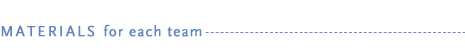• copy of "Lever Loads" student handout (PDF or HTML)
• two rulers, 30 cm (12 in.) plastic or lightweight wood
• pencil
• five weights (such as washers or coins)
• tape
• sheet of paper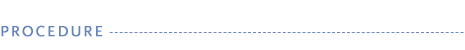1. Let students investigate how levers can be used to lift objects in this activity.

2. Distribute materials and copies of the "Lever Loads" student handout to pairs of students.

3. In Part I students will discover that the amount of force needed to lift a load changes depending on where the fulcrum is placed.

4. In Part II students repeat the investigation, this time comparing the distance the load is lifted to the distance they push down the other lever arm.

5. After completing the activity, ask students to think about how NOVA team members used levers to raise the two-ton obelisk. Where did they place the fulcrum (the pivot point) in relationship to the obelisk (load)? Why did the team need to pack stone and earth underneath the lever to support it?

6. Archimedes (287-212 B.C.E.) understood levers well enough to claim, "Give me a lever long enough and a place to stand, and I could lift the world." Discuss this quote with students. What does Archimedes mean by a lever "long enough"? Where might he place the fulcrum?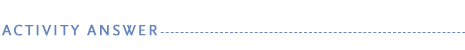In Part I, students will notice that the closer the fulcrum is to the load, the less force is required to lift the load. There is a point where the fulcrum is so close to the load that it appears no force is required at all. The weight of the lever itself (in this case, the ruler) is actually providing the needed force. This is one reason why crowbars are very heavy.

In Part II, students should note that the position of the fulcrum also affects how far you push down and how far the load is lifted up. Levers trade force (how hard you push) for distance (how far you move the load). For example, when you apply a small force over a long distance, you move the load a small distance.

Students should notice that when they use less force to lift the load, the distance they push the lever arm down is larger than the distance the load is lifted. When they use more force to lift the load, the distance they push the lever arm is smaller than the distance the load is lifted. When the fulcrum is placed in the middle of the lever, the distance the load is lifted is about equal to the distance that the other end of the lever is pushed down. In this situation there is essentially no mechanical advantage—the distance over which the force is applied equals the distance the load is lifted.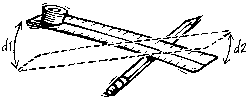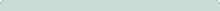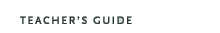Secrets of Lost Empires I—Obelisk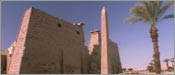Original broadcast:February 12, 1997